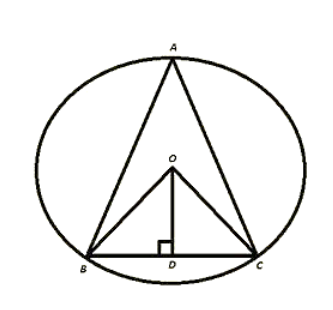# O is the circumference of the triangle ABC and OD is perpendicular on BC. Prove that ∠BOD = ∠A.

Question:

O is the circumference of the triangle ABC and OD is perpendicular on BC. Prove that ∠BOD = ∠A.

Solution:

Given O is the circum centre of triangle ABC and OD⊥BC

To prove ∠BOD = 2∠A

Proof:

In ΔOBD and ΔOCD

∠ODB = ∠ODC [Each 90°]

OB = OC [Radius of circle]

OD = OD [Common]

Then ΔOBD ≅ ΔOCD    [By RHS Condition].

∴ ∠BOD = ∠COD  .... (i)   [PCT].

By degree measure theorem

∠BOC = 2∠BAC

⇒ 2∠BOD = 2∠BAC [By using (i)]

⇒ ∠BOD = ∠BAC.